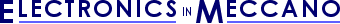www.eleinmec.com

The 555 Astable Circuit
By Tim Surtell

In an astable circuit, the output continually switches between the high and low states without any intervention from the user, producing a 'square' wave.  An astable has no stable state - hence the name "astable".

This type of circuit could be used to give a mechanism intermittent motion by switching a motor on and off at regular intervals.  It can also be used to flash lamps and LEDs, and is useful as a 'clock' pulse for other digital ICs and circuits.

An astable circuit can be constructed using a 555 timer IC and a few other components.  To work out the values of these components, we first need to understand the terminology used to describe the square wave output of an astable.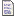Related Articles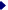The 555 Timer - Background information about the 555The 555 Monostable CircuitThe 555 Bistable Circuit

#### Waveform Terminology

##### Frequency

Figure 1 shows the square wave output of an astable circuit.  There is one 'cycle' (one high period and one low period) every 1/10th second, so the wave has a 'period' of 0.1 seconds.  The time period is directly related to the 'frequency' of the wave by the equation...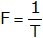...where F is the frequency of the wave in Hertz (Hz) and T is the time period of the wave in seconds.  Therefore, the wave in figure 1 has a frequency of 10Hz...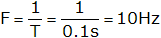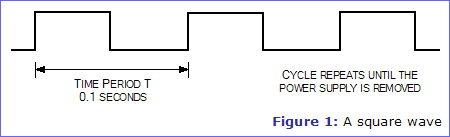##### Duty Cycle

Figure 2 shows another square wave output from an astable circuit.  Like the wave in figure 1, it also has a frequency of 10Hz, but this time the high and low periods are unequal.  The high period, also known as the 'mark' period, is 0.025 seconds long.  The low period, also known as the 'space' period, is 0.075 seconds long.  The ratio of these periods is known as the 'mark-to-space ratio'.  In this case it is 1:3, but it is usually expressed as a percentage called the 'duty cycle', which in this case is 25%.  This simply means that the output is high for 25% of each cycle and low for 75% of each cycle.  The duty cycle of the square wave in figure 1 would be 50%.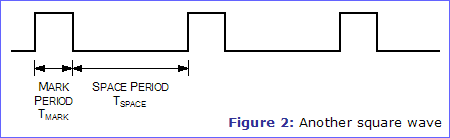#### The 555 Astable Circuit

Figure 3 shows the circuit diagram of the 555 astable circuit.  Notice that the resistor values R1 and R2, and the capacitor value C are unspecified.  The values of these components determine the frequency and duty cycle of the astable, and they may be calculated using the equations below...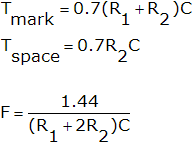...where Tmark and Tspace are the mark and space periods in seconds, F is the frequency in Hertz, and R1, R2, and C are the component values in Ohms (Ω) and Farads (F).

The third equation is in fact derived from the first two, but we will only consider using the first two equations.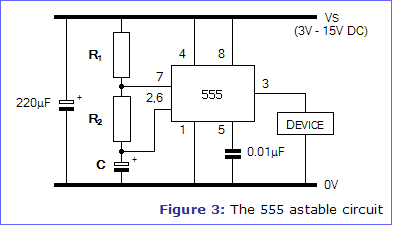##### Doing the Calculations

Here is a step-by-step guide to calculating the component values - an example is given in curly braces {}.

1. Firstly, decide on the time Tspace that you require.  This can be very small (milliseconds) or large (minutes), but it must be expressed in seconds.  {I choose Tspace = 1 second}
2. Next, guess a value for the capacitor C, expressed in Farads.  For starters, try 10μF.  {I choose C = 10μF}
3. Put the values of Tspace and C into the equation below and calculator resistor R2...4. Finally, decide on the time Tmark that you require {I choose Tmark = 1.5 seconds} and use the equation below to calculate resistor R1...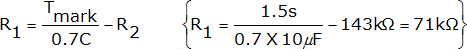You should now have the component values required to build the astable circuit in figure 3.  However, if any of the resistor values calculated are smaller than 1kΩ or larger than 1MΩ, you should re-do the calculations with a different value for capacitor C until you get resistor values within the acceptable range.

Note that you cannot get Tspace and Tmark to be exactly equal (i.e. a 50% duty cycle), but you can get them approximately equal.  Ensure that R2 is at least 10kΩ in step 3, and then omit step 4 and make R1 much smaller than R2 - for example, the minimum value of 1kΩ.

##### Varying the Frequency

If you will need to adjust the frequency of the astable circuit in use, you can use a linear variable resistor for R1, as shown in figure 4.

Because the resistance of a variable resistor goes down to around 0Ω at one end of its range, a 1kΩ resistor is placed in series with it so that the value of R1 will never fall below 1kΩ. If R2 is much larger than 1kΩ, this means that at the lowest shaft setting of the variable resistor Tspace and Tmark will be approximately equal. As the shaft is turned, Tspace will become longer.

If your chosen variable resistor has three connections, it is a potentiometer, and you should connect to the centre connection and either of the end connections.Further InformationResistors - Includes information about variable resistors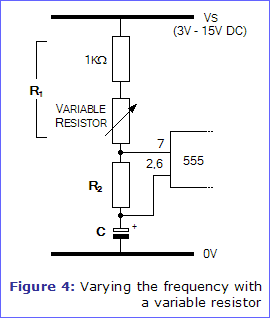#### Building the Circuit

A stripboard layout for the 555 astable circuit is given in figure 5.  It provides a direct output from the 555 (pin 3), but if you want the astable to control another device, such as a motor, you will probably want to leave some extra board space for any extra components required.  Some suggestions are given in "Using the 555 output" from part 1 of this series, The 555 Timer.

The 220μF capacitor is included to smooth the power supply, and can be omitted if you are using a regulated supply or the circuit functions correctly without it.

##### Construction
1. Cut a piece of stripboard to 12 tracks x 24 columns + extra columns for any extra components required.
2. Fit the 6 wire links.
3. Fit the resistors R1 and R2.
4. Fit the 8-pin IC socket, taking care to align the notch as shown in the layout.  Do not insert the 555 at this time.
5. Fit the 0.01μF, 220μF capacitors and timing capacitor C.  Ensure that the polarity of the 220μF capacitor is correct.  The leads will be marked with '+' or '-'.  The timing capacitor you have chosen could also be polarised, and should also be carefully placed.
6. Fit the components associated with the device that the 555 is controlling.
7. Insert the 555 into its socket, taking care to align the notch with the notch in the socket.
8. Cut the copper tracks where an X is shown.
9. Connect up a 3V - 15V DC power supply and test the circuit.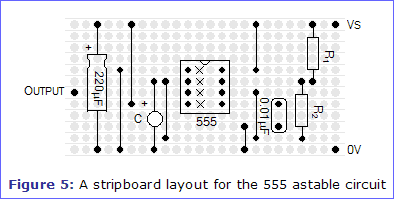Article Information
Source: Electronics in Meccano - www.eleinmec.com | First published in EiM: Issue 3 (March 1999)Hosted on a Memset Dedicated Server | © 1998 - 2023 Tim Surtell